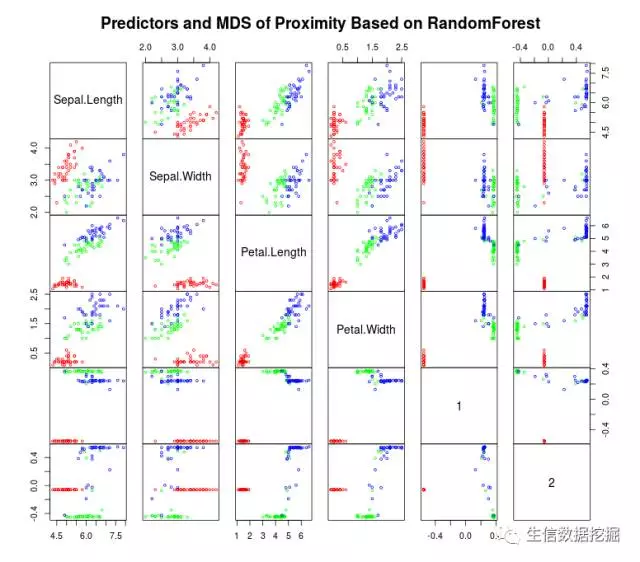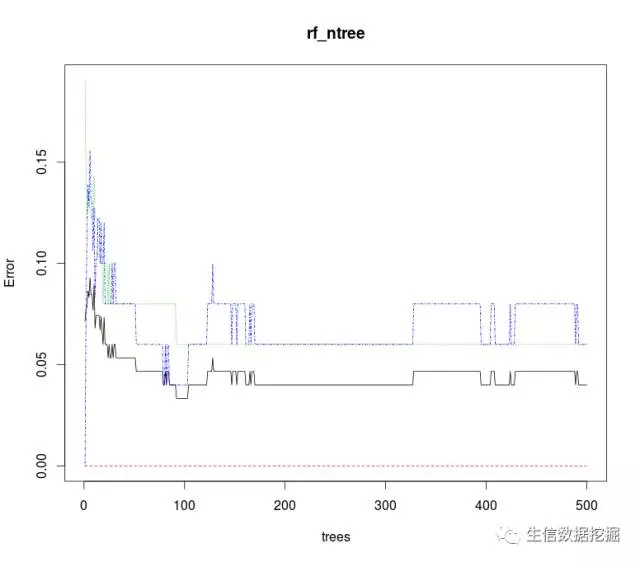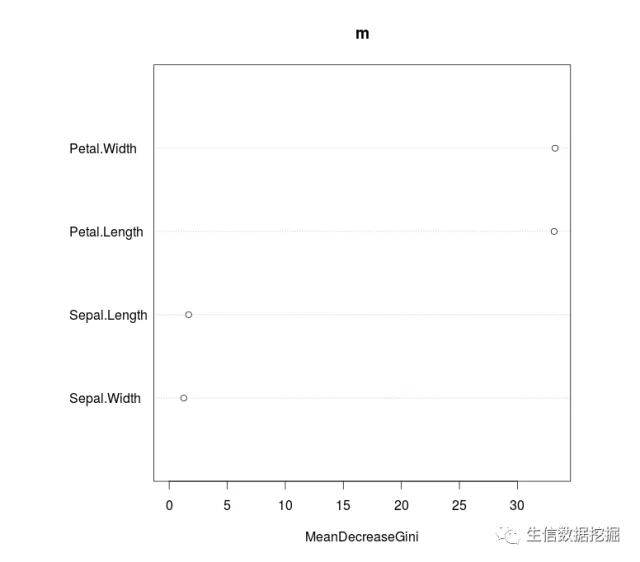• 数据导入数据处理建立模型模型评估更内容关注公众号：邯郸路220号子彬院 导入数据 导入pandas,并且重命名为pd。 import pandas as pd #通过互联网读取泰坦尼克乘客档案，并存储在变量titanic中。 titanic = pd....
文章目录导入数据导入pandas,并且重命名为pd。数据导入数据处理建立模型模型评估更多内容关注公众号：邯郸路220号子彬院
导入数据
导入pandas,并且重命名为pd。
import pandas as pd
#通过互联网读取泰坦尼克乘客档案，并存储在变量titanic中。
titanic = pd.read_csv( ‘titanic.txt’)
＃引入pandas，并且重命名为pd。
将熊猫作为pd导入
＃通过互联网读取泰坦尼克乘客档案，并存储在变量titanic中。
泰坦尼克号= pd.read_csv（‘titanic.txt’）
数据导入
#导入pandas,并且重命名为pd。
import pandas as pd
#通过互联网读取泰坦尼克乘客档案，并存储在变量titanic中。
titanic= pd.read_csv('http://biostat.mc.vanderbilt.edu/wiki/pub/Main/DataSets/titanic.txt')

#人工选取pclass、age以及sex作为判别乘客是否能够生还的特征。
x = titanic[['pclass','age','sex']]
y = titanic['survived']

数据处理
#对于缺失的年龄信息,我们使用全体乘客的平均年龄代替，这样可以在保证顺利训练模型的同时,尽可能不影响预测任务。
x['age'].fillna(x['age'].mean(), inplace= True)

C:\ProgramData\Anaconda3\lib\site-packages\pandas\core\generic.py:5434: SettingWithCopyWarning:
A value is trying to be set on a copy of a slice from a DataFrame

See the caveats in the documentation: http://pandas.pydata.org/pandas-docs/stable/indexing.html#indexing-view-versus-copy
self._update_inplace(new_data)

#对原始数据进行分割，258的乘客数据用于测试。
from sklearn.cross_validation import train_test_split
x_train, x_test, y_train, y_test = train_test_split (x, y,test_size=0.25,random_state = 33)

C:\ProgramData\Anaconda3\lib\site-packages\sklearn\cross_validation.py:41: DeprecationWarning: This module was deprecated in version 0.18 in favor of the model_selection module into which all the refactored classes and functions are moved. Also note that the interface of the new CV iterators are different from that of this module. This module will be removed in 0.20.
"This module will be removed in 0.20.", DeprecationWarning)

#对类别型特征进行转化,成为特征向量。
from sklearn.feature_extraction import DictVectorizer
vec = DictVectorizer (sparse = False)
x_train = vec.fit_transform(x_train.to_dict (orient= 'record') )
x_test = vec.transform(x_test.to_dict(orient= 'record'))

建立模型
使用多种用于评价分类任务性能的指标,在测试数据集上对比单一决策树(DecisionTree)、随机森林分类器(RandomForestClassifier)以及梯度提升决策树(Gradient Tree Boosting)的性能差异。
#使用单-决策树进行模型训练以及预测分析。
from sklearn.tree import DecisionTreeClassifier
dtc = DecisionTreeClassifier()
dtc.fit(x_train, y_train)
dtc_y_pred= dtc.predict(x_test)

#使用随机森林分类器进行集成模型的训练以及预测分析。
from sklearn. ensemble import RandomForestClassifier
rfc = RandomForestClassifier()
rfc.fit(x_train, y_train)
rfc_y_pred = rfc.predict(x_test)

#使用梯度提升决策树进行集成模型的训练以及预测分析。
from sklearn.ensemble import GradientBoostingClassifier
gbc = GradientBoostingClassifier ()
gbc.fit(x_train, y_train)
gbc_y_pred = gbc.predict (x_test)

模型评估
#从sklearn .metrics导人classification report。
from sklearn.metrics import classification_report
#输出单一决策树在测试集上的分类准确性，以及更加详细的精确率、召回率、F1指标。
print('The accuracy of decision tree is', dtc.score(x_test, y_test))
print(classification_report(dtc_y_pred, y_test))
#输出随机森林分类器在测试集上的分类准确性，以及更加详细的精确率、召回率、F1指标。
print('The accuracy of random forest classifier is', rfc.score(x_test, y_test))
print(classification_report(rfc_y_pred, y_test))
#输出梯度提升决策树在测试集上的分类准确性，以及更加详细的精确率、召回率、F1指标。
print('The accuracy of gradient tree boosting is', gbc.score(x_test, y_test))
print(classification_report(gbc_y_pred, y_test))

The accuracy of decision tree is 0.7811550151975684
precision    recall  f1-score   support

0       0.91      0.78      0.84       236
1       0.58      0.80      0.67        93

avg / total       0.81      0.78      0.79       329

The accuracy of random forest classifier is 0.78419452887538
precision    recall  f1-score   support

0       0.90      0.78      0.84       233
1       0.60      0.79      0.68        96

avg / total       0.81      0.78      0.79       329

The accuracy of gradient tree boosting is 0.790273556231003
precision    recall  f1-score   support

0       0.92      0.78      0.84       239
1       0.58      0.82      0.68        90

avg / total       0.83      0.79      0.80       329

输出表明:在相同的训练和测试数据条件下,仅仅使用模型的默认配置，梯度上升决策树具有最佳的预测性能,其次是随机森林分类器,最后是单一决策树。
更多内容关注公众号：邯郸路220号子彬院


展开全文• 原文链接：来自公众号生信数据挖掘 目录R实现随机森林随机森林R包估值过程oob errorR randomForest函数实现安装程序包，查看样本数据...如上图所示， 随机森林模型包含个树形分类器，预测结果由分类器（决策树
原文链接：来自公众号生信数据挖掘
目录R实现随机森林随机森林R包估值过程袋外错误率（oob error）R randomForest函数实现安装程序包，查看样本数据结构建模与观察
R实现随机森林

该文只简单的讲解关于的R的随机森林具体实现步骤，只简单介绍了随机森林，未对原理进行过多解释

随机森林模型是一种预测能力较强的数据挖掘模型，常用于进行分类预测和数据回归分析，这里我们只讨论分类预测。

使用的数据集是R自带的 iris 的数据集如上图所示， 随机森林模型包含多个树形分类器，预测结果由多个分类器（决策树）投票得出，每个决策树相当于一个大师，通过自己在数据集中学到的知识对于新的数据进行分类。
在随机森林算法的函数randomForest()中有两个非常重要的参数，而这两个参数又将影响模型的准确性，它们分别是mtry和ntree。一般对mtry的选择是逐一尝试，直到找到比较理想的值，ntree的选择可通过图形大致判断模型内误差稳定时的值。

随机森林R包
randomForest::randomForest  该包中主要涉及5个重要函数，关于这5个函数的语法和参数请见下方：
randomForest()： 此函数用于构建随机森林模型
randomForest(formula, data=NULL, …, subset,na.action=na.fail)

formula：指定模型的公式形式，类似于y~x1+x2+x3…;
data：指定分析的数据集；
.ntree：指定随机森林所包含的决策树数目，默认为500；
mtry：指定节点中用于二叉树的变量个数，默认情况下数据集变量个数的二次方根（分类模型）或三分之一（预测模型）。一般是需要进行人为的逐次挑选，确定最佳的m值；

估值过程

指定m值，即随机产生m个变量用于节点上的二叉树，m的选择原则是使错误率最低。
应用bootstrap自助法在原数据集中又放回地抽取k个样本集，组成k棵决策树，每个决策树输出一个结果。
对k个决策树组成的随机森林对样本进行分类或预测：分类原则：少数服从多数；预测原则：简单平均。

袋外错误率（oob error）
如何选择最优的特征个数m，要解决这个问题，我们主要依据计算得到的袋外错误率.
在构建每棵树时，对训练集使用了不同的bootstrap sample（随机且有放回地抽取）。所以对于每棵树而言，部分训练实例没有参与这棵树的生成，它们称为第k棵树的oob样本
袋外错误率（oob error）计算方式如下：

对每个样本计算它作为oob样本的树对它的分类情况
以简单多数投票作为该样本的分类结果
最后用误分个数占样本总数的比率作为随机森林的oob误分率

R randomForest函数实现
在R语言中，我们调用randomForest包中的randomForest()函数来实现随机森林算法。
安装程序包，查看样本数据结构
#R package#

install.packages("randomForest")

library(randomForest)#选取训练样本(70%)和测试样本(30%)

index <- sample(2,nrow(iris),replace = TRUE,prob=c(0.7,0.3))

traindata <- iris[index==1,]

testdata <- iris[index==2,]

遍历比较确定最优mtry值。mtry参数是随机森林建模中，构建决策树分支时随机抽样的变量个数。选择合适的mtry参数值可以降低随机森林模型的预测错误率。示例的数据中共有4个自变量，可通过遍历设定mtry参数1至4进行4次建模，并打印出每次建模的错误率，选择错误率最低的mytry取值。
- 选择最优mtry参数值
n <- ncol(iris) -1

errRate <- c(1)

for (i in 1:n){

m <- randomForest(Species~.,data=iris,mtry=i,proximity=TRUE)

err<-mean(m$err.rate) errRate[i] <- err } print(errRate)  0.05462878 0.04320072 0.04302654 0.04316091#选择平均误差最小的m m= which.min(errRate) print(m)  输出结果： 3 根据遍历打印结果，当mtry=3时，错误率达到最低，因此本次建模过程中以3作为mtry参数值 选择合适的ntree参数值: ntree参数指出建模时决策树的数量。ntree值设置过低会导制错误率偏高，ntree值过高会提升模型复杂度，降低效率。以mtry=3进行随机森林建模，并将模型错误率与决策树数量的关系可视化，如下： - 选择最优ntree参数值  rf_ntree <- randomForest(Species~.,data=iris) plot(rf_ntree)  结果图如下：从图中可以看到，当ntree=100时，模型内的误差就基本稳定了，出于更保险的考虑，我们确定ntree值为100。 建模与观察 根据以上结果，以mtry=3,mtree=100为参数建立随机森林模型，并打印模型信息 m <- randomForest(Species~.,data=traindata,mtry=3, ntree=100, proximity=TRUE) print(m)  结果如下所示： Call: randomForest(formula = Species ~ ., data = traindata, mtry = 3, ntree = 100, proximity = TRUE) Type of random forest: classification Number of trees: 100No. of variables tried at each split: 3 OOB estimate of error rate: 5.41% Confusion matrix: setosa versicolor virginica class.error setosa 39 0 0 0.00000000 versicolor 0 33 3 0.08333333 virginica 0 3 33 0.08333333  MDSplot()：函数用于实现随机森林的可视化 specialmds <- cmdscale(1 - m$proximity, eig=TRUE)

op <- par(pty="s")

pdf("trees_proximity.pdf")

pairs(cbind(traindata[,1:length(traindata)-1], specialmds$points), cex=0.6, gap=0,col=c("red", "green", "blue")[as.numeric(traindata$Species)], main=":Predictors and MDS of Proximity Based on RandomForest")

par(op)

print(specialmds$GOF) dev.off()importance()函数：用于计算模型变量的重要性 importance(m)  结果：  MeanDecreaseGini Sepal.Length 1.201101 Sepal.Width 1.685455 Petal.Length 32.926760 Petal.Width 37.559478  绘制重要性图示： varImpPlot(m)从返回的数据和图形可知，在四个变量中，Petal.Width和Petal.Length最为重要，其次分别是Sepal.Length和Sepal.Width. - 接着使用已建立好的随机森林模型进行新数据集的测试 pred <- predict(m,newdata=testdata)   展开全文机器学习 R语言 • 前面已经发了一个版本了，但是那个看着是二分类，估计很多人也不太好下手改，因为有人问，我就好事做到底吧，来一个多分类吧，大家还可以参考上一篇SVM的更新自己实现一下大影像的分类，我这就不搞重复的了，先上... • ## 随机森林r语言实现（超详细） 万次阅读 多人点赞 2017-12-25 11:49:29 随机森林就是用随机的方式建立一个森林，森林里面有很的决策树，并且每棵树之间是没有关联的。得到一个森林后，当有一个新的样本输入，森林中的每一棵决策树会分别进行一下判断，进行类别归类（针对分类算法），... 随机森林算法介绍 算法介绍： 简单的说，随机森林就是用随机的方式建立一个森林，森林里面有很多的决策树，并且每棵树之间是没有关联的。得到一个森林后，当有一个新的样本输入，森林中的每一棵决策树会分别进行一下判断，进行类别归类（针对分类算法），最后比较一下被判定哪一类最多，就预测该样本为哪一类。 随机森林算法有两个主要环节：决策树的生长和投票过程。 决策树生长步骤： 从容量为N的原始训练样本数据中采取放回抽样方式(即bootstrap取样)随机抽取自助样本集，重复k（树的数目为k）次形成一个新的训练集N，以此生成一棵分类树； 每个自助样本集生长为单棵分类树，该自助样本集是单棵分类树的全部训练数据。设有M个输入特征，则在树的每个节点处从M个特征中随机挑选m(m < M)个特征，按照节点不纯度最小的原则从这m个特征中选出一个特征进行分枝生长，然后再分别递归调用上述过程构造各个分枝，直到这棵树能准确地分类训练集或所有属性都已被使用过。在整个森林的生长过程中m将保持恒定； 分类树为了达到低偏差和高差异而要充分生长，使每个节点的不纯度达到最小，不进行通常的剪枝操作。 投票过程： 随机森林采用Bagging方法生成多个决策树分类器。 基本思想： 给定一个弱学习算法和一个训练集，单个弱学习算法准确率不高，可以视为一个窄领域专家； 将该学习算法使用多次，得出预测函数序列，进行投票，将多个窄领域专家评估结果汇总，最后结果准确率将大幅提升。 随机森林的优点： 可以处理大量的输入变量; 对于很多种资料，可以产生高准确度的分类器; 可以在决定类别时，评估变量的重要性; 在建造森林时，可以在内部对于一般化后的误差产生不偏差的估计; 包含一个好方法可以估计遗失的资料，并且，如果有很大一部分的资料遗失，仍可以维持准确度; 提供一个实验方法，可以去侦测 variable interactions; 对于不平衡的分类资料集来说，可以平衡误差; 计算各例中的亲近度，对于数据挖掘、侦测偏离者（outlier）和将资料视觉化非常有用; 使用上述。可被延伸应用在未标记的资料上，这类资料通常是使用非监督式聚类。也可侦测偏离者和观看资料; 学习过程很快速。 缺点 随机森林已经被证明在某些噪音较大的分类或回归问题上会过拟合; 对于有不同级别的属性的数据，级别划分较多的属性会对随机森林产生更大的影响，所以随机森林在这种数据上产出的属性权值是不可信的。 R语言实现 寻找最优参数mtry，即指定节点中用于二叉树的最佳变量个数 library("randomForest") n<-length(names(train_data)) #计算数据集中自变量个数，等同n=ncol(train_data) rate=1 #设置模型误判率向量初始值 for(i in 1:(n-1)){ set.seed(1234) rf_train<-randomForest(as.factor(train_data$IS_LIUSHI)~.,data=train_data,mtry=i,ntree=1000)
rate[i]<-mean(rf_train$err.rate) #计算基于OOB数据的模型误判率均值 print(rf_train) } rate #展示所有模型误判率的均值 plot(rate)  寻找最佳参数ntree，即指定随机森林所包含的最佳决策树数目 set.seed(100) rf_train<-randomForest(as.factor(train_data$IS_LIUSHI)~.,data=train_data,mtry=12,ntree=1000)
plot(rf_train)    #绘制模型误差与决策树数量关系图
legend(800,0.02,"IS_LIUSHI=0",cex=0.9,bty="n")
legend(800,0.0245,"total",cex=0.09,bty="n")

随机森林模型搭建
set.seed(100)
rf_train<-randomForest(as.factor(train_data$IS_LIUSHI)~.,data=train_data,mtry=12,ntree=400,importance=TRUE,proximity=TRUE)  importance设定是否输出因变量在模型中的重要性，如果移除某个变量，模型方差增加的比例是它判断变量重要性的标准之一； proximity参数用于设定是否计算模型的临近矩阵； ntree用于设定随机森林的树数。 输出变量重要性:分别从精确度递减和均方误差递减的角度来衡量重要程度。 importance<-importance(rf_train) write.csv(importance,file="E:/模型搭建/importance.csv",row.names=T,quote=F) barplot(rf_train$importance[,1],main="输入变量重要性测度指标柱形图")
box()

提取随机森林模型中以准确率递减方法得到维度重要性值。type=2为基尼系数方法
importance(rf_train,type=1)

varImpPlot(x=rf_train,sort=TRUE,n.var=nrow(rf_train$importance),main="输入变量重要性测度散点图")  信息展示 print(rf_train) #展示随机森林模型简要信息 hist(treesize(rf_train)) #展示随机森林模型中每棵决策树的节点数 max(treesize(rf_train));min(treesize(rf_train)) MDSplot(rf_train,train_data$IS_OFF_USER,palette=rep(1,2),pch=as.numeric(train_data$IS_LIUSHI)) #展示数据集在二维情况下各类别的具体分布情况  检测 pred<-predict(rf_train,newdata=test_data) pred_out_1<-predict(object=rf_train,newdata=test_data,type="prob") #输出概率 table <- table(pred,test_data$IS_LIUSHI)
sum(diag(table))/sum(table)  #预测准确率
plot(margin(rf_train,test_data\$IS_LIUSHI),main=观测值被判断正确的概率图)

randomForest包可以实现随机森林算法的应用，主要涉及5个重要函数，语法和参数请见下
1:randomForest()函数用于构建随机森林模型
randomForest(formula, data=NULL, ..., subset, na.action=na.fail)
randomForest(x, y=NULL, xtest=NULL, ytest=NULL, ntree=500,
mtry=if (!is.null(y) && !is.factor(y))
max(floor(ncol(x)/3), 1) else floor(sqrt(ncol(x))),
replace=TRUE, classwt=NULL, cutoff, strata,
sampsize = if (replace) nrow(x) else ceiling(.632*nrow(x)),
nodesize = if (!is.null(y) && !is.factor(y)) 5 else 1,
maxnodes = NULL,
importance=FALSE, localImp=FALSE, nPerm=1,
proximity, oob.prox=proximity,
norm.votes=TRUE, do.trace=FALSE,
keep.forest=!is.null(y) && is.null(xtest), corr.bias=FALSE,
keep.inbag=FALSE, ...)

formula指定模型的公式形式，类似于y~x1+x2+x3…;
data指定分析的数据集；
subset以向量的形式确定样本数据集；
na.action指定数据集中缺失值的处理方法，默认为na.fail，即不允许出现缺失值，也可以指定为na.omit，即删除缺失样本；
x指定模型的解释变量，可以是矩阵，也可以是数据框；
y指定模型的因变量，可以是离散的因子，也可以是连续的数值，分别对应于随机森林的分类模型和预测模型。这里需要说明的是，如果不指定y值，则随机森林将是一个无监督的模型；
xtest和ytest用于预测的测试集；
ntree指定随机森林所包含的决策树数目，默认为500；
mtry指定节点中用于二叉树的变量个数，默认情况下数据集变量个数的二次方根（分类模型）或三分之一（预测模型）。一般是需要进行人为的逐次挑选，确定最佳的m值；
replace指定Bootstrap随机抽样的方式，默认为有放回的抽样
classwt指定分类水平的权重，对于回归模型，该参数无效；
strata为因子向量，用于分层抽样；
sampsize用于指定样本容量，一般与参数strata联合使用，指定分层抽样中层的样本量；
nodesize指定决策树节点的最小个数，默认情况下，判别模型为1，回归模型为5；
maxnodes指定决策树节点的最大个数；
importance逻辑参数，是否计算各个变量在模型中的重要性，默认不计算，该参数主要结合importance()函数使用；
proximity逻辑参数，是否计算模型的临近矩阵，主要结合MDSplot()函数使用；
oob.prox是否基于OOB数据计算临近矩阵；
norm.votes显示投票格式，默认以百分比的形式展示投票结果，也可以采用绝对数的形式；
do.trace是否输出更详细的随机森林模型运行过程，默认不输出；
keep.forest是否保留模型的输出对象，对于给定xtest值后，默认将不保留算法的运算结果。
2:importance()函数用于计算模型变量的重要性
importance(x, type=NULL, class="NULL", scale=TRUE, ...)

x为randomForest对象；
type可以是1，也可以是2，用于判别计算变量重要性的方法，1表示使用精度平均较少值作为度量标准；2表示采用节点不纯度的平均减少值最为度量标准。值越大说明变量的重要性越强；
scale默认对变量的重要性值进行标准化。
3:MDSplot()函数用于实现随机森林的可视化
MDSplot(rf, fac, k=2, palette=NULL, pch=20, ...)

rf为randomForest对象，需要说明的是，在构建随机森林模型时必须指定计算临近矩阵，即设置proximity参数为TRUE；
fac指定随机森林模型中所使用到的因子向量（因变量）；
palette指定所绘图形中各个类别的颜色；
pch指定所绘图形中各个类别形状；
还可以通过R自带的plot函数绘制随机森林决策树的数目与模型误差的折线图
4:rfImpute()函数可为存在缺失值的数据集进行插补（随机森林法），得到最优的样本拟合值
rfImpute(x, y, iter=5, ntree=300, ...)
rfImpute(x, data, ..., subset)

x为存在缺失值的数据集；
y为因变量，不可以存在缺失情况；
iter指定插值过程中迭代次数；
ntree指定每次迭代生成的随机森林中决策树数量；
subset以向量的形式指定样本集。
5:treesize()函数用于计算随机森林中每棵树的节点个数
treesize(x, terminal=TRUE)

x为randomForest对象；
terminal指定计算节点数目的方式，默认只计算每棵树的根节点，设置为FALSE时将计算所有节点（根节点+叶节点）。
一般treesize()函数生成的结果用于绘制直方图，方面查看随机森林中树的节点分布情况。

展开全文r语言 集成算法
• 随机森林个决策树的集成学习，每个决策树用bagging的方法选数据集，并且在选择最佳属性划分的时候随机划分一些属性进行分类，比单个分类器效果更好，泛化能力更强。 代码解释 1.用结构体的嵌套实现树的结构。 2....
随机森林
随机森林是多个决策树的集成学习，每个决策树用bagging的方法选数据集，并且在选择最佳属性划分的时候随机划分一些属性进行分类，比单个分类器效果更好，泛化能力更强。
代码解释
1.用结构体的嵌套实现树的结构。
2.makerandomtree递归的创建树。
3.可自动适应不同的类别标签，不同的属性个数和不同的类别个数。
4.函数ent（D）返回D的信息熵。
代     码
树的主体：
function tree=makerandomtree(D,a)
tree=struct('isnode',1,'a',0.0,'mark',0.0,'child',{});%isnode判断是否是分支还是叶子，a表示节点属性，若节点是叶子，a表示分类结果，child是孩子
tree(1).a=1;%给tree分配一个确切的内存
if length(unique(D(:,end)))==1%D中样本属于同一类别
tree.isnode=0;%把tree标记为树叶
tree.a=D(1,end);%把tree的类别标记为D的类别
return
end
if sum(a)==0 ||length(D)==0 %属性划分完毕
tree.isnode=0;%把tree标记为树叶
tree.a=mode(D(:,end));%把tree的类别标记为D出现最多的类别
return
end
for i=1:length(a)
if a(i)==1
if length(unique(D(:,i)))==1
tree.isnode=0;%把tree标记为树叶
tree.a=mode(D(:,end));%把tree的类别标记为D出现最多的类别
return
end
end
end
k=ceil(log2(sum(a)));%随机选k个属性进行学习
randomindices=zeros(length(a),1); %随机去掉属性的索引，结束之后要恢复随机去掉的属性
if k>1
i=1;
su=sum(a);
while 1 %随机去掉一些属性，使得剩下的属性是k个
random1=randperm(length(a),1);
if a(random1)==1
randomindices(random1)=1;
a(random1)=0;
i=i+1;
end
if i==(su-k+1)
break;
end
end
end

gain=zeros(length(a),1); %保存每个属性的信息增益
best=zeros(length(a),1); %保存每个属性的最佳划分

for i=1:length(a)
if a(i)==1
t=D(:,i);
t=sort(t);

gain1=zeros(length(t)-1,1);
for j=1:length(t)-1%二分划分
ta=(t(j)+t(j+1))/2;

Df=D(D(:,i)<=ta,:);
Dz=D(D(:,i)>ta,:);
gain1(j)=ent(D)-(ent(Df)*length(Df(:,end))/length(D(:,end))+ent(Dz)*length(Dz(:,end))/length(D(:,end)));
end

[gain(i),j]=max(gain1);
ta=(t(j)+t(j+1))/2;
best(i)=ta;
end
end
[~,m]=max(gain);%选择信息增益最大的属性
D1=D(D(:,m)<=best(m),:);
D2=D(D(:,m)>best(m),:);
a(m)=0;
for i=1:length(a)  %恢复随机去掉的属性
if randomindices(i)==1
a(i)=1;
end
end
tree.a=best(m); %建立分支
tree.mark=m;
% disp('****************************')
% tree.a
% tree.mark
tree.isnode=1;
tree.child(1)=makerandomtree(D1,a);
tree.child(2)=makerandomtree(D2,a);

end

计算ent
function f=ent(D)%计算信息商
l=unique(D(:,end));
if length(D)==0
f=0;
return
end
f=0;
t=zeros(length(l),1);
for i=1:length(D(:,end))
for j=1:length(l)
if D(i,end)==l(j)
t(j)=t(j)+1;
break;
end
end
end
n=length(D(:,end));
for i=1:length(l)
f=f+(t(i)/n)*log2(t(i)/n);
end
f=-f;
end

主函数
function randomforest()
clc
clear all
T=3;%bagging采样的次数
M = importdata('D:\毕业设计\数据集1\australian.txt');  %读取数据
[sm,sn]=size(M);
% for i=1:sm             %归一化
%     mins=min(M(i,1:sn-1));
%     maxs=max(M(i,1:sn-1));
%     for j=1:sn-1
%         M(i,j)=2*(M(i,j)-mins)/(maxs-mins)-1;
%     end
% end
indices=crossvalind('Kfold',M(1:sm,sn),10); %十折交叉，划分训练集和测试集
testindices=(indices==1); %测试集索引
trainindices=~testindices;%训练集索引
trainset=M(trainindices,:); %获取训练集
testset=M(testindices,:);%获取测试集
[testm,~]=size(testset);
[trainm,trainn]=size(trainset);

predict=zeros(trainm,T);
for t=1:T %开始bagging采样
D=[];%训练集
for i=1:trainm%采样
k=randperm(trainm,1);
D=[D;trainset(k,:)];
end
[~,sn]=size(D);
a=ones(sn-1,1);%属性集合a，1代表该属性未被划分

tree=makerandomtree(D,a);%递归构造简单决策树

for i=1:trainm
treet=tree;
while 1

if treet.isnode==0
predict(i,t)=treet.a;
break;
end
if trainset(i,treet.mark)<=treet.a
treet=treet.child(1);
else
treet=treet.child(2);
end

end
end

end
acc=0;
for i=1:trainm
if trainset(i,end)==mode(predict(i,:))
acc=acc+1;
end
end
acc=acc/trainm
end




展开全文• ## python实现随机森林

万次阅读 多人点赞 2018-08-26 18:10:59
随机森林指的是利用棵决策树对样本进行训练并预测的一种分类器。可回归可分类。 所以随机森林是基于颗决策树的一种集成学习算法，常见的决策树算法主要有以下几种： 1. ID3：使用信息增益g(D,A)进行特征选择 ...
• 机器学习算法（8）python实现随机森林分类） 可以把随机森林看成是决策树的集 合。随机森林背后的逻辑是对分别受较大方差影响的个决策树取平均值， 以建立一个具有更好的泛化性能和不易过拟合的强大模型。 ...python 机器学习 决策树
• 随机森林：对UCI数据集的分类实现 关于随机森林的原理网上有许许多，这里不再赘述。sklearn中的RandomForestClassifier包即可满足你对随机森林的调用。 UCI数据集可在UCI官方页面中进行下载并使用。 本文主要用于...机器学习 python 可视化
• 为提高图像分类性能，提出了一种图像分类方法。其基本思想是将图像内容的不确定性描述看作是一个随机过程，采用...最后，构造随机森林分类器，实现模糊熵特征向量的分类。实验结果表明，该方法的错分率低，分类耗时少。
• 第六章 多分类、决策树分类、随机森林分类 第七章 分类评估、聚类 第八章 密度聚类、谱聚类 第九章 深度学习、TensorFlow安装和实现 第十章 TensorFlow深入、TensorBoard 十一章 DNN深度神经网络手写图片识别 十二章...
• 第六章 多分类、决策树分类、随机森林分类 第七章 分类评估、聚类 第八章 密度聚类、谱聚类 第九章 深度学习、TensorFlow安装和实现 第十章 TensorFlow深入、TensorBoard 十一章 DNN深度神经网络手写图片识别 十二章...
• 在上一篇（https://blog.csdn.net/baymax_007/article/details/82748544）中，利用逻辑回归实现资讯多分类。本文在之前基础上，又引入决策树、随机森林、多层感知分类器、xgboost和朴素贝叶斯分类算法，并对验证集和...逻辑回归 决策树 xgboost
• 随机森林顾名思义，是用随机的方式建立一个森林，森林里面有很的决策树组成，随机森林的每一棵决策树之间是没有关联的。在得到森林之后，当有一个新的输入样本进入的时候，就让森林中的每一棵决策树分别进行一下...
• 随机森林（randomforest）是一种利用分类树对数据进行判别与分类的方法，它在对数据进行分类的同时，还可以给出各个变量（基因）的重要性评分，评估各个变量在分类中所起的作用。 随机森林是一个最近比较火的...机器学习 算法 C++
• 随机森林是一个包含个决策树的分类器，该分类器的输出结果是由所有决策树输出结果的众数而定， 每个决策树都生长于一个被称为bootstrap样本的训练数据集之上，所谓“bootstrap样本”的构造原理为： 对于容量为n...
• 在讲随机森林前，我先讲一下什么是集成学习。集成学习通过构建并结合分类器来完成学习任务。集成学习通过将个学习器进行结合，常可获得比单一学习器更好的泛化性能。 考虑一个简单例子：在二分类任务中，假定...python 机器学习
• 随机森林算法实现对iris数据集的分类 随机森林主要应用于回归和分类两种场景，又侧重于分类随机森林是指利用棵树对样本数据 进行训练、分类并预测的一种方法。它在对数据进行分类的同时，还可以给出各个变量的...
• 我开始构建一个有趣的随机森林实现，但最终决定这可能是Java中一个不错的小型机器学习库的开始。 我的重点将放在易于理解的代码上，而不是性能上。 该死的东西似乎还不错。 与scikit-learn相比，我的测试具有相同或...
• 1.决策树与随机森林都属于机器学习中监督学习的范畴，主要用于分类问题。 决策树算法有这几种：ID3、C4.5、CART，基于决策树的算法有bagging、随机森林、GBDT等。 决策树是一种利用树形结构进行决策的算法，对于样本...
• 前面的博客讲到装袋(bagging)是一种一次性综合个基本分类器的预测结果，进行多数表决(分类)或取均值(回归)的组合算法。 装袋又称自助聚集：以均匀概率有放回从原训练数据集中挑选出与原...随机森林(Random For...
• R语言实现 随机森林算法介绍 算法介绍 简单的说随机森林就是用随机的方式建立一个森林 森林里面有很的决策树 并且每棵树之间是没有关联的 得到一个森林后 当有一个新的样本输入 森林 中的每一棵决策树会分别进行...
• R 语言实现 随机森林算法介绍 算法介绍 简单的说随机森林就是用随机的方式建立一个森林森林里面有很的决策 树并且每棵树之间是没有关联的得到一个森林后当有一个新的样本输入 森林中的每一棵决策树会分别进行一下...
• 随机森林和决策树一样既可以做分类又可以做回归（更用来做分类问题） 随机森林是使用集成学习的思想将颗决策树集成一种学习器的算法，中间利用到bagging的投票思想，少数服从多数进行集成学习 随机森林的随机...决策树 python 机器学习 算法
• MapReduce编程方式实现分类模型以P2P流量识别为例，分别与单个随机森林和集成其他算法进行对比，实验表明提出模型能获得更好的P2P流量识别综合分类性能，该模型也为二类型分类提供了一种可行的参考方法。集成学习 MapReduce P2P流量识别
• 在机器学习中，随机森林是一个包含个决策树的分类器， 并且其输出的类别是由个别树输出的类别的众数而定。 Leo Breiman和Adele Cutler发展出推论出随机森林的算法。 而 “Random Forests” 是他们的商标。 这个...Spark
• 基于Bagging的集成学习：随机森林的原理及其实现引入Bagging装袋随机森林随机森林分类随机森林回归python实现随机森林分类随机森林回归 引入 “三个臭皮匠赛过诸葛亮”——弱分类器组合成强分类器。 Q1.什么是随机...
• 最近在研究TLD算法，在做目标...随机森林，指的是利用棵树对样本进行训练并预测的一种分类器。该分类器最早由Leo Breiman和Adele Cutler提出，并被注册成了商标。简单来说，随机森林就是由棵CART（Classification A计算机视觉 算法 应用
• 随机森林指的是利用棵树对样本进行训练并预测的一种分类随机森林的原理 在机器学习中，随机森林是一个包含个决策树的分类器 根据下列算法而建造每棵树： 用N来表示训练用例（样本）的个数，M表示特征数目。 ...决策树 机器学习 python...